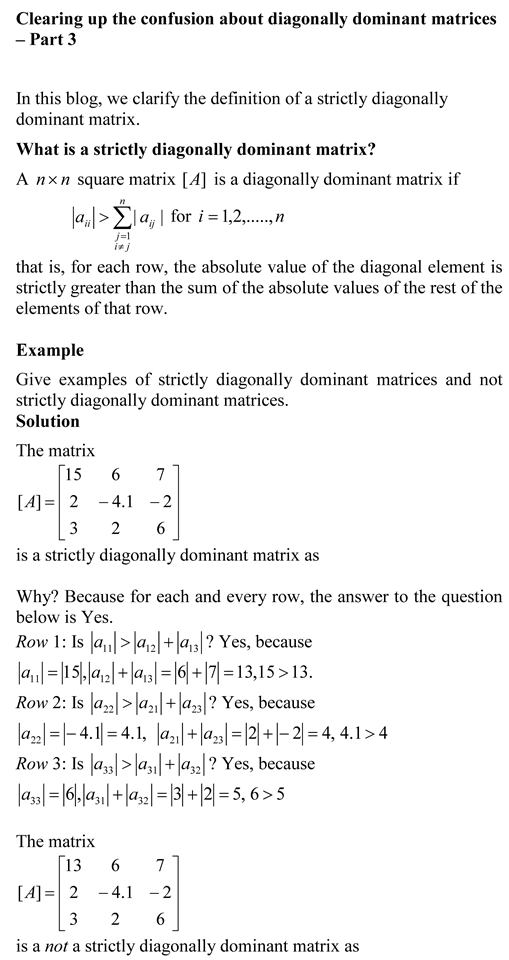## Clearing up the confusion about diagonally dominant matrices – Part 3You can view the above document as a pdf file as well.

Other blogs on diagonally dominant matrices
Clearing up the confusion about diagonally dominant matrices – Part 1

Clearing up the confusion about diagonally dominant matrices – Part 2

_________________________________________________________

This post is brought to you by

• Holistic Numerical Methods Open Course Ware:
• the textbooks on
• the Massive Open Online Course (MOOCs) available at

## Clearing up the confusion about diagonally dominant matrices – Part 2

In a previous post, we discussed the confusion about the definition and associated properties of diagonally dominant matrices.  In this blog, we answer the next question.

What is a weak diagonally dominant matrix?

The answer is simple – the definition of a weak(ly) diagonally dominant matrix is identical to that of a diagonally dominant matrix as the inequality used for the check is a weak inequality of greater than or equal to (≥).  See the previous post on Clearing up the confusion about diagonally dominant matrices – Part 1 where we define a diagonally dominant matrix.

Other blogs on diagonally dominant matrices
Clearing up the confusion about diagonally dominant matrices – Part 1

_______________________________________

This post is brought to you by

• Holistic Numerical Methods Open Course Ware:
• the textbooks on
• the Massive Open Online Course (MOOCs) available at

## MOOC on Introduction to Matrix Algebra released

Introduction to Matrix Algebra is available as a MOOC now on Udemy. Of course, it is free! https://www.udemy.com/matrixalgebra/.  You will have a lifetime access to 177 lectures, 14+ hours of high quality content, 10 textbook chapters complete with multiple choice questions and their complete solutions.

Learning Objectives are

• know vectors and their linear combinations and dot products
• know why we need matrix algebra and differentiate between various special matrices
• carry unary operations on matrices
• carry binary operations on matrices
• differentiate between inconsistent and consistent system of linear equations via finding rank of matrices
• differentiate between system of equations that have unique and infinite solutions
• use Gaussian elimination methods to find solution to a system of equations
• use LU decomposition to find solution to system of equations and know when to choose the method over Gaussain elimination
• use Gauss-Seidel method to solve a system of equations iteratively
• find quantitatively how adequate your solution is through the concept of condition numbers
• find eigenvectors and eigenvalues of a square matrix

_____________________________________________________

This post is brought to you by

## Friday October 31, 2014, 11:59PM EDT, November 1, 2014 3:59AM GMT – Release Date for an Opencourseware in Introduction to Matrix Algebra

In a true Netflix style, on Halloween night, Friday October 31, 2014 at 11:59PM EST, we are releasing all resources simultaneously for an open courseware on Introduction to Matrix Algebra athttp://mathforcollege.com/ma/.  The courseware will include

• 150 YouTube video lectures of total length of approximately 14 hours,
• 10 textbook chapters,
• 10 online multiple-choice quizzes with complete solutions,
• 10 problem sets, and
• PowerPoint presentations.

So set your calendar for October 31 for some matrix algebra binging rather than candy binging.  For more info and questions, contact Autar Kaw.Chapter 1: Introduction

Chapter 2: Vectors

Chapter 3: Binary Matrix Operations

Chapter 4: Unary Matrix Operations

Chapter 5: System of Equations

Chapter 6: Gaussian Elimination Method

Chapter 7: LU Decomposition

Chapter 8: Gauss-Seidel Method

Chapter 10: Eigenvalues and Eigenvectors

________________________
This post is brought to you by

## Misconceptions about diagonal and tridiagonal matrices

A reader wrote: “I purchased on lulu the 2nd edition of your Introduction to Matrix Algebra for self study, and the book just arrived. I started reading it and found some annoying errors. For example on Chapter 1, page 5: for the first (diagonal) matrix, why is there a zero located in a33, when you defined on the previous page that only diagonal entries of square matrix can be non-zero (this answer is different on your free online pdf) . Also, on page 6 for the tridiagonal matrix, why is there a zero located in the diagonal below the major diagonal? I was wondering if you can provide me with the list of errors and corrections, because it’s going to be very difficult to study the material on my own and the errors in the book just makes it more frustrating.”

My answer: “There is no erratum issued yet on the book.

A diagonal matrix is diagonal based on the nondiagonal elements being zero. The diagonal elements have no restrictions. They can be zero or nonzero.

A tridiagonal matrix is a square matrix in which all elements not on the following are zero – the major diagonal, the above the major diagonal, and the diagonal below the major diagonal. The major diagonal, the diagonal above the major diagonal, and the diagonal below the major diagonal have no restrictions. They can be zero or nonzero.

The concerns you have raised are some of the common misconceptions students develop about these special matrices.

______________________________________________

This post is brought to you by Holistic Numerical Methods: Numerical Methods for the STEM undergraduate at http://nm.MathForCollege.com, the textbook on Numerical Methods with Applications available from the lulu storefront, the textbook on Introduction to Programming Concepts Using MATLAB, and the YouTube video lectures available at http://nm.MathForCollege.com/videos. Subscribe to the blog via a reader or email to stay updated with this blog. Let the information follow you.